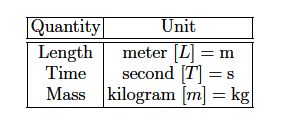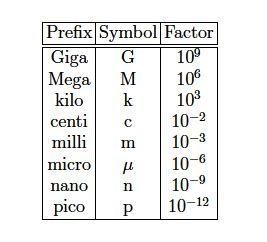Physics

# What are Units in Mathematical Tools?

Physical quantities are measured using instruments; a meter stick for length (L), a clock for time (T), and a balance scale for mass (m). Thus, physical quantities have magnitudes and units. The fundamental units in the international System of Units [SI] are:For example, we write for distance d = 4.3 m, for time t = 76.4 s, or for mass m = 0.56 kg. Prefixes are used for small and large values:Thus, 3.4 nm = 3.4 x 10-9 m and 0.34 μs = 3.4 x 10-7 s.

In any equation, the units on the left and right side must be equal: dimensional analysis. For example, we have distance [L], time [T], and speed [LIT]. We use dimensional analysis to check whether an equation is possibly correct For the formula x = ½ vt2, we have [L] = ([L]/[T])[T]2 = [L][T] . That is, equation x = ½ vt2 is incorrect. For x = ½ vt we get [L] L ([L]/[T])[T] = [L], so that the equation x = ½ vt might be correct. Dimensional analysis does not tell you anything about factors such as ½, π, etc.

The argument of mathematical functions [sine, cosine, the other trigonometric functions, exponential function, logarithm, etc] are always dimensionless. Thus, for the equation x = A cos Bt, we have [Bt] = 1 so that [B] = 1/[t] = 1/s, and [A] = [x] = m.